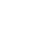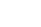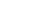## Chemistry Paper 1 Questions and Answers - Arise and Shine Mock Exams 2022

Instructions to candidates:

• Write your Name and Index Number in the spaces provided.
• Sign and write the date of examination in the spaces provided above.
• Answer ALL questions in spaces provided.
• ALL working must be shown clearly where necessary.
• Mathematical tables and silent non-programmable calculators may be used.

For Examiner’s Use Only

 Questions Maximum Score Candidate’s Score 1 -30 80

### Questions

1.
1. Draw a labeled diagram showing the structure ofion (2 marks)
2. Explain why the atom is said to be electricity neutral. (1 mark)
2. Explain why luminous flame is yellow and sooty. (2 marks)
3. The diagrams below represent a simplified industrial process for manufacturing hydrochloric acid. Study it and answer the question that follows.1. Give two sources of substance X (1 mark)
2. State the role of the substance labeled Z. (1 mark)
3. State two uses of dilute hydrochloric acid. (1 mark)
4. During laboratory preparation of oxygen reagent H is added to Sodium Peroxide.
1. Name the equation for the reaction that takes place. (1 mark)
2. Write the equation for the reaction that takes place. (1 mark)
3. Name a gas that is mixed with oxygen to be used in welding. (1 mark)
5. The elements fluorine, Chlorine, Bromine and Iodine belong to group (VII). Select the element with lowest melting point, Give a reason. (2 marks)
6. Starting with magnesium granules, describe how you can suitably obtain magnesium hydroxide solid. (3 marks)
7. Aqueous Copper (II) Sulphate was electrolyzed using graphite electrodes.
1. How does PH of the electrolyte change during electrolysis? (1 mark)
2. Write the cathode equation. (1 mark)
3. The experiment was repeated using copper electrodes. Write the anode equation. (1 mark)
8. In an experiment, dry hydrogen gas was passed Overheated magnesium oxide as shown in the diagram below.1. State and explain the observations made in the combustion tube. (1 mark)
2. The experiment was repeated using Lead (II) oxide. State the observations made in the combustion tube. (1 mark)
9. Explain why an increase in temperature increases the rate of a reaction. (2 marks)
10. 10g of an oxide of Sodium contains 5.9g of sodium. Its molar mass is 78. Determine its molecular formula. (Na = 23, O = 16) (3 marks)
11. Study the flow chart below and answer the questions that follow:1. Identify substance B and C (1 mark)
2. Name and draw the structure of substance A (1 mark)
3. Write the equation for the reaction that occur when D react with excess Bromine gas in presence of sunlight. (1 mark)
12. A piece of sodium metal was placed in a beaker containing pure water.
1. Write the equation for the reaction that occurs. (1 mark)
2. Using oxidation numbers show that the reaction in (a) above is redox. (2 marks)
13.
1. The set up in the figure below can be used to prepare dry nitrogen (iv) oxide. Use it to answer the questions that follow.1. Name the substance G and Q (1 mark)
2. Complete the set up to show how nitrogen (iv) oxide is exposed to air. (1 mark)
2. State the observation made when a gas jar containing nitrogen (II) oxide is exposed to air. (1 mark)
14. The table below shows ammeter readings recorded when 2M potassium hydroxide and 2M aqueous ammonia were tested separately.
 Electrolyte Current (A) 2M Potassium Hydroxide 8.1 2M Ammonia 2.5
Explain the difference in the ammeter readings. (2 marks)
15. Compound H has the following structure1. Give the name of the compound H. (1 mark)
2. In which group of compounds does H belong? (1 mark)
3. Name the reagents that were used to prepare compound H. (1 mark)
16.
1. State Boyle’s law. (1 mark)
2. Study the set up below and answer the questions that follow.State and explain the observations made. (2 marks)
17. Draw a well labeled diagram that can be used to electroplate iron spoon with silver. (3 marks)
18. 2g of sodium hydroxide is added to 40cm3 of 1M sulphuric (vi) acid. What volume of 0.1M potassium hydroxide solution will be needed to neutralize the excess acid?
(Na = 23.0 O = 16.0 H = 1.0) (3 marks)
19.
1. Explain why it is not advisable to prepare a sample of carbon (iv) oxide using calcium carbonate and dilute sulphuric (vi) acid. (2 marks)
2. Give one use of coke. (1 mark)
20. The table below shows the standard electrode potentials of the elements P and Q.
Half reactions                    EɵV
P2+(aq) + 2e → P(s)         -0.44
Q2+(aq) + 2e  → Q(s)       -2.37
Use the information to predict whether the reaction represented below can take place. (2 marks)
P(s) + Q2+(aq) →  P2+(aq) + Q(s)
21. The table below shows the atomic numbers and electron affinities of three elements. The letters are not actual chemical symbols. Use it to answer the questions that follow.
 Element Atomic number Electron Affinity kJ/mol A 17 -349 kJ/mol B 35 -325 kJ/mol C 53 -295kJ?mol
1. What is electron affinity? (1 mark)
2. Explain the trend in electron affinity from A to C. (2 marks)
22. A sample of herbicide in solution form is suspected to contain Lead (II) ions. Describe how the presence of Lead (II) ions can be established. (2 marks)
23. A monomer has the following structure.1. Draw the structure of the polymer that contain three monomers. (1 mark)
2. A sample of the polymer formed from the monomer has a molecular mass of 7740. Determine the number of monomers that formed the polymer.
(C = 12.0 H = 1.0 O = 16.0) 2 marks)
24.
1. The figure below is an incomplete diagram that can be used to illustrate how alpha, beta and gamma radiations can be distinguished from each otherComplete the diagram above (1½ marks)
2. Radioactive decay ofgives, gamma radiations and X.
1. Identify X. (½ mark)
2. Write a nuclear equation for the decay. (1 mark)
25. A small amount of sulphur was burnt in a deflagrating spoon. The burning sulphur was then lowered into a gas jar full of oxygen.
1. The product formed is dissolved in water. Suggest the PH of the resulting solution. Give a reason. (1 mark)
2. Explain the observation made when pink flower is immediately dropped in the solution obtained in (a) above. (2 marks)
26. The table below gives three experiment on the reaction of excess dilute hydrochloric acid and 0.5g of zinc done under different conditions. In each the change in mass of the reactant was recorded at different time intervals.
 Experiment Forms od Zinc Hydrochloric acid I Powder 0.6M II Granules 0.6M III Powder 1M
On the axis below, draw and label the three curves that could be obtained from such results. (3 marks)27. The relative atomic mass of an element is 10.28, it has two isotopes 10R and 11R. Calculate the relative abundance of each isotope. (2 marks)
28.
1. Define the standard enthalpy of formation of a substance. (1 mark)
2. Use the thermochemical equations below to answer the questions that follow.
H2(g) + ½ O2(g) → H2O(1) ΔH = -286kJ/mol
C(s) + ½ O2(g) →  C O2(g) ΔH = -393kJ/mol
C4 H10(g) + 9/2 O2(g) →  4CO2 + 5H2O(1) ΔH = -2877kJ/mol
1. Draw an energy cycle diagram linking the heat of formation of butane with its heat of combustion of its constituent elements. (2 marks)
2. Calculate the heat of formation of butane. (1 mark)
29. Name an appropriate apparatus that can be used to measure 29.3cm3 of 0.1M sodium hydroxide solution in the laboratory. (1 mark)
30. Copper is mostly extracted from copper pyrite.
1. Give the chemical formula of copper pyrite. (1 mark)
2. State the role of silica that is added during extraction of copper from copper pyrite. (1 mark)

### MARKING SCHEME

1.
1.2. The number of Protons and electrons in an atom are equal.√
2. It contains hot glowing Carbon Particles ✓1 which on cooling form soot ✓1
3.
1. Cracking of long chain alkanes.
• Electrolysis of brine.
• Reacting natural gas with steam
(Mark the first two)
2. Increases the Surface area over which hydrogen chloride gas dissolves ✓1
3.
• Removing rust from metal
• Sewage treatment / Treatment of water
• Making dyes, drugs and photographic material
• PH control and neutralization in industries
(mark the first two for ½ mark each)
4.
1. Water ✓1
2. 2 Na₂ O₂ + 2H2O(L) → 4 NaOH(aq) + O2(g)√
Penalize ½mark for missing or wromg state symbols
3. Ethyne / Acetylene
-Hydrogen OR √ Penalize fully if not balanced.
5. Flourine ✓1
It has the smallest molecular size ✓½ hence weakest intermolecular forces✓½ / few van der weal forces.
6. Add excess Magnesium granules✓½ to dilute HCl/HNO3/H2SO4,✓½ Stir and Filter,✓½ to the filtrate add Sodium hydroxide/Potassium hydroxide/Ammonia solution,✓½ filter✓½ and dry the residue between filter papers
7.
1. PH of the electrolyte decreases,✓ there is accumulation of Hydrogen ions (H+)✓ since Copper (II) ions are discharged at the cathode.
2. Cu2+ (aq) + 2e → Cu(s) ✓ (1 mark)
3. Cu(s) → Cu2+ (aq) + 2e ✓ (1 mark)
8.
1. White magnesium oxide remains white✓½/ same Magnesium is very high the reactivity series hence hydrogen cannot reduce✓½ magnesium oxide.
9. Increase in temperature increases the kinetic energy✓½ of the reacting particle, this results in increase in number of successful✓1 collision / effective collision hence increases rate of reaction✓½
10.11.12.
1. 2Na(s) + 2H2O(l) → 2NaOH(aq) + H2(g) ✓ (1 mark)
2. The oxidation number of Sodium metal increases from 0 to +1, it is oxidized
Oxidation number of Hydrogen in water decreases of +1 to 0✓ it is reduced
13.
1.
1. G - Anhydrous Calcium Chloride✓½                        do not accept calcium oxide
2. Q - Concentrated nitric (V) acid
3.2. Red - brown fumes produced✓½ nitrogen (II) oxide is oxidized✓½ to nitrogen (IV) oxide by air.
14. Potassium hydroxide is fully ionized✓½/ strong base hence has higher√1/2 electrical conductivity, while Ammonia solution is partially✓½ ionized / weak base hence lower √1/2 electrical conductivity
15.
1. Methylbutanoate ✓1
2. Esters ✓1
3. Methanol✓½ Not tied
Butanoic acid ✓½
16.
1. The volume of a fixed mass of inversely proportional to its pressure AT A constant temperature✓½
2. Moist red litmus paper turns blue✓½ then red✓½ Ammonium chloride dissociate when heated into Ammonia gas and Hydrogen Chloride gas.✓½ Ammonia diffuses faster than hydrogen chloride gas
17.Labeling 2 marks
Workability 1mark.
Anode must be Silver and electrolyte must have silver ions.
18.19.
1. Calcium Carbonate react with dilute sulphuric (vi) acid to form Calcium sulphate that is insoluble✓ and forms a coating and stops further reaction
(Accept equation for 1 mark)
2.
1. Used as a reducing agent in extraction of metals
2. Used as fuel
(Mark the first one for 1 mark).
20. The e.m.f for the reaction is
= -2.37 - (-0.44)
= -1.83V✓ 1 mark
The reaction cannot take place since the E value is negative. ✓1 mark
21.
1. Electron affinity. It is the amount of energy released when an atom gain election(s)
2. Electron affinity decreases from A to C.✓1 The atomic radius increases down ✓½ the group hence the tendency of ✓½ Atoms of A to C to gain electrons also decreases.
22. To a sample of the herbicide in test tube, add Potassium Iodide solution,✓1 A yellow precipitate is formed√1 OR To a solution of the herbicide in a test tube add dilute hydrochloric acid or any soluble Chloride✓½ , and warm✓½ the mixture. A white precipitate is formed✓½ that is soluble on warming. ✓½
23.
1.2. Molecular mass of monomer = 48 + 32 + 6 = 86✓½
Number of Monomers = 7740/86 ✓1 = 90✓½
24.
1.2.25.
1. PH values ranging from 4.5 - 6 ✓½ NB State one value within the range penalize if range is given
Sulphur (IV) oxide dissolves in water forming Sulphuric (IV) acid✓½ which is a weak acid.
2. Pink flower is bleached! ✓1
Sulphuric (IV) acid removes oxygen✓1 atom in the dye, and form Sulphuric (VI) acid/Sulphuric (IV) acid bleaches by reduction.
26.27.28.
1. It is the enthalpy change that occurs when a substance is formed from its constituent elements under standard conditions. 1. ✓½29. Burette✓1/reject any other
OR
Syringe
30.
1. CuFeS2 ✓1
2. Silica react with Iron (11) oxide to form Iron (II) silicate which is a major component of slag.

• ✔ To read offline at any time.
• ✔ To Print at your convenience
• ✔ Share Easily with Friends / Students